# Java Do While Loop With Examples

iterations do not have a fixed value, it is recommended to use a do-while loop as it is guaranteed to be executed once. This is possible because the condition is checked post the body of the loop is executed. That is why it is an Exit Control Loop

Thus, do while Java is a variant of while loop that runs the code block before the condition is evaluated as true and then repeat it as long as the condition is true, just like while loop.

## Do While Java Syntax

do{

//body of the code

}while(condition);

Here the condition is a Boolean expression that appears at the end of the loop. If the expressions are evaluated to true, the control jumps back to the do statement, and the loop is executed again. The process repeats till the Boolean expression is evaluated as false.

Example

public class Example {

public static void main(String[] args) {

int i=1;

do{

System.out.println(i);

i++;

}while(i<=5);

}

}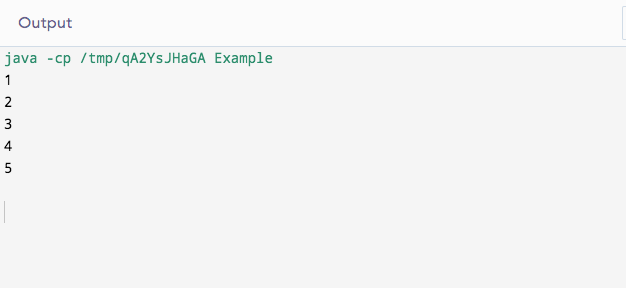Explanation: The given loop runs six times. Hence, the value of i is 6, but it is not printed as the condition evaluates to false.

## How Does a Do-While Loop Execute?

1. The control falls into the do while the Java loop as ‘do’ is encountered.
2. The statements in the body of the loop (code) are executed.
3. The variable is updated.
4. The flow now comes to
5. the condition.
6. If it is true, then step 6 is executed; otherwise, the flow goes out of the loop.
7. Flow moves back to step 2

## Applications

### Do While Java Infinite

An infinite loop is created when the Boolean expression is passed as true in the do-while java loop.

Here is a do-while Java infinite loop example.

public class Example {

public static void main(String[] args)  {

do {

System.out.println(“Start Processing inside do while loop”);

// Any other statements can be added

System.out.println(“End Processing of do while loop”);

} while (true);

}

}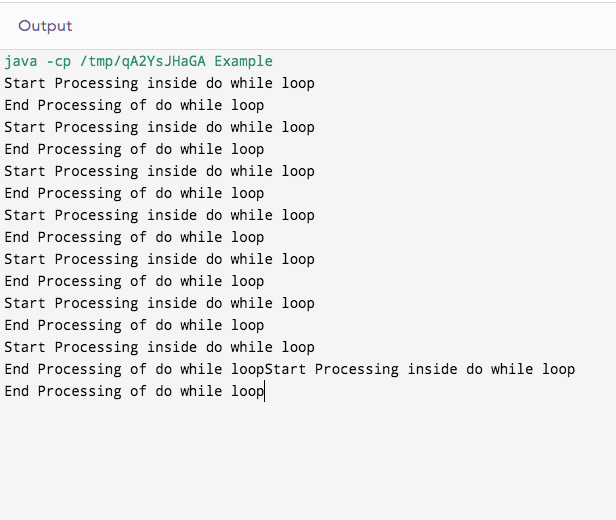Explanation: The statements keep on being executed till the program is terminated using the IDE.

### Sum of Natural Numbers up to a Given Number

public class Example {

public static void main(String args[])

int x = 7, sum = 0;

do { // The line will be printed even

// if the condition is false

sum += x;

x–;

} while (x > 0);

System.out.println(“Sum: ” + sum);

}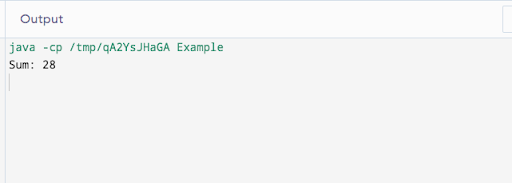### Iteration of Array using Do While Java Loop.

Here’s an example of iteration of an integer array using do-while loop in Java:

class Example{

public static void main(String args[]){

int arr[]={0,1,45,9};

int i=0;

do{

System.out.println(arr[i]);

i++;

}while(i<4);

}

}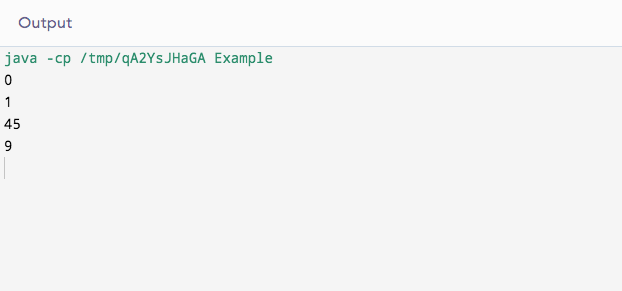## Nesting of Do-While Loops

It is possible to have a do-while in a do-while Java loop. This is known as nesting of do-while construction. There is no upper bound to the depth of nesting. A do-while can have any construct like if, while, switch, etc., inside it. For example:

class Example{

public static void main(String args[])

{

int a=10;

do   // 1st do while

{

System.out.println(10);

do// 2nd do while

System.out.println(20);

}

while(false);

}

while(false);

}

}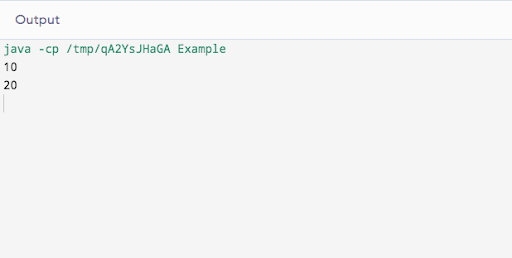## Do While vs While Loop

• While loop is an entry control loop, whereas the do-while is an exit controlled loop
• Java while looks cleaner than do while Java loop.
• The Do while loop executes at least once irrespective of the condition.

### Why use Do-While?

When you require your program to be executed a minimum once, use do-while. For example, you have to take input from the user until the user enters a negative number. In such a case, a do-while loop is used as the initial input can be positive or negative, but we require input. In all other cases, it is easier to use a while loop.

## Things to Remember

1. The body of the do-while loop is required to have a minimum of one statement
2. The condition (boolean expression) at the end of the loop must always result in a Boolean value.
3. Without the condition, the loop cannot be executed.
4. An error would be raised if a do statement does not correspond with a while statement.

## Conclusion

The do-while Java loop is used for iterating a set of statements until a given condition is met. In this blog, you learned about the loop, its syntax, uses, nesting, and comparison with the while loop.

If you’re interested to learn more about JAVA, Full-stack software development, check out upGrad & IIIT-B’s Executive PG Program in Full-stack Software Development which is designed for working professionals and offers 500+ hours of rigorous training, 9+ projects, and assignments, IIIT-B Alumni status, practical hands-on capstone projects & job assistance with top firms.

## Land on Your Dream Job

UPGRAD AND IIIT-BANGALORE'S PG DIPLOMA IN FULL STACK
ENROLL TODAY

## 0 replies on “Java Do While Loop With Examples”